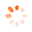Home / Products

# DESCRIPTION

Technical Description

Fundamentals of fluid mechanics include Bernoulli's principle and the continuity equation. The continuity equation states that the flow velocity in a steady, incompressible and friction-free flow is inversely proportional to the cross-sectional area. The sum of the static and dynamic pressure is constant in a steady flow, according to Bernoulli's principle. A change in the cross-sectional area leads to a corresponding change in the static pressure. These physical laws make it possible to calculate the dynamic pressure and the flow velocity of an incompressible fluid in a steady flow.

Using the Venturi tube HM 220.01 in the experimental plant HM 220 allows the continuity equation and Bernoulli's equation to be clearly and practically evaluated and applied. An in-depth understanding of the laws is promoted by means of illustrative experiments.

The accessory is placed in the measuring section to generate a Venturi-shaped cross-sectional profile of the flow. The static pressure is measured via various pressure measuring points along the measuring section and read on the manometer. The difference to the total pressure is the dynamic pressure.

The well-structured instructional material sets out the fundamentals and provides a step-by-step guide through the experiments.

Learning Objectives / Experiments

- examination of the continuity equation and Bernoulli's principle
- determination of the dynamic pressure
- calculation of the flow velocity
- representation of the pressure curve as a function of the cross-sectional area

# Subscribe now